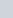Define an identity.
Question:

Define an identity.

Solution:

An identity is an equation which is true for all values of the variable (s).

For example,

$(x+3)^{2}=x^{2}+6 x+9$

Any number of variables may involve in an identity.

An example of an identity containing two variables is

$(x+y)^{2}=x^{2}+2 x y+y^{2}$

The above are all about algebraic identities. Now, we define the trigonometric identities.

An equation involving trigonometric ratios of an angle(say) is said to be a trigonometric identity if it is satisfied for all valued offor which the trigonometric ratios are defined.

For examples,

$\sin ^{2} \theta+\cos ^{2} \theta=1$

$1+\tan ^{2} \theta=\sec ^{2} \theta$

$1+\cot ^{2} \theta=\operatorname{cosec}^{2} \theta$